# 五、支持向量机

## 线性支持向量机分类

SVM 的基本思想能够用一些图片来解释得很好，图 5-1 展示了我们在第 4 章结尾处介绍的鸢尾花数据集的一部分。这两个种类能够被非常清晰，非常容易的用一条直线分开（即线性可分的）。左边的图显示了三种可能的线性分类器的判定边界。其中用虚线表示的线性模型判定边界很差，甚至不能正确地划分类别。另外两个线性模型在这个数据集表现的很好，但是它们的判定边界很靠近样本点，在新的数据上可能不会表现的很好。相比之下，右边图中 SVM 分类器的判定边界实线，不仅分开了两种类别，而且还尽可能地远离了最靠近的训练数据点。你可以认为 SVM 分类器在两种类别之间保持了一条尽可能宽敞的街道（图中平行的虚线），其被称为最大间隔分类。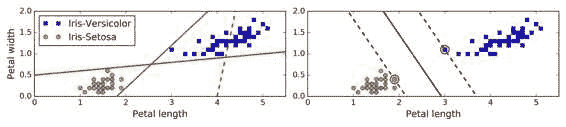SVM 对特征缩放比较敏感，可以看到图 5-2：左边的图中，垂直的比例要更大于水平的比例，所以最宽的“街道”接近水平。但对特征缩放后（例如使用 Scikit-Learn 的 StandardScaler），判定边界看起来要好得多，如右图。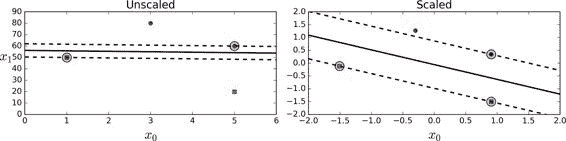### 软间隔分类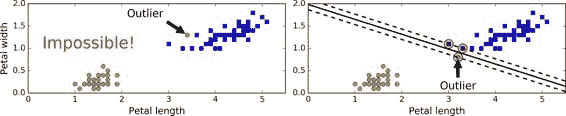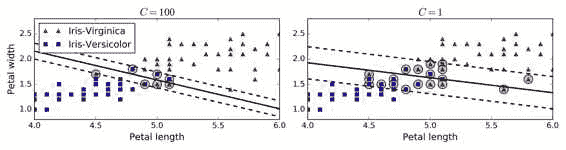import numpy as np
from sklearn import datasets
from sklearn.pipeline import Pipeline
from sklearn.preprocessing import StandardScaler
from sklearn.svm import LinearSVC

X = iris["data"][:, (2, 3)] # petal length, petal width
y = (iris["target"] == 2).astype(np.float64) # Iris-Virginica

svm_clf = Pipeline((
("scaler", StandardScaler()),
("linear_svc", LinearSVC(C=1, loss="hinge")),
))

svm_clf.fit(X, y)

Then, as usual, you can use the model to make predictions:

>>> svm_clf.predict([[5.5, 1.7]])
array([ 1.])


LinearSVC要使偏置项规范化，首先你应该集中训练集减去它的平均数。如果你使用了StandardScaler，那么它会自动处理。此外，确保你设置loss参数为hinge，因为它不是默认值。最后，为了得到更好的效果，你需要将dual参数设置为False，除非特征数比样本量多（我们将在本章后面讨论二元性）

## 非线性支持向量机分类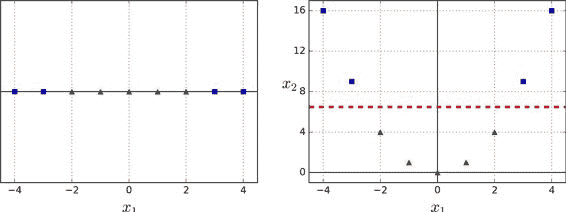from sklearn.datasets import make_moons
from sklearn.pipeline import Pipeline
from sklearn.preprocessing import PolynomialFeatures

polynomial_svm_clf = Pipeline((
("poly_features", PolynomialFeatures(degree=3)),
("scaler", StandardScaler()),
("svm_clf", LinearSVC(C=10, loss="hinge"))
))

polynomial_svm_clf.fit(X, y)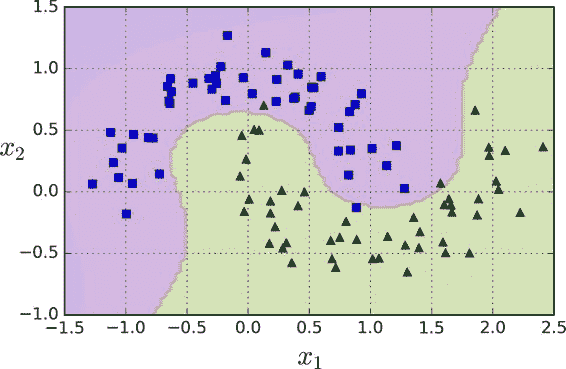### 多项式核

from sklearn.svm import SVC
poly_kernel_svm_clf = Pipeline((
("scaler", StandardScaler()),
("svm_clf", SVC(kernel="poly", degree=3, coef0=1, C=5))
))
poly_kernel_svm_clf.fit(X, y)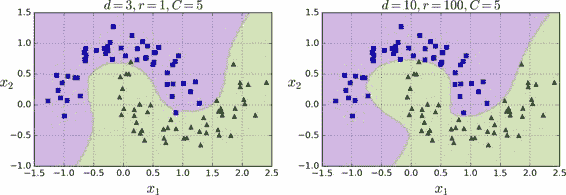### 增加相似特征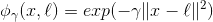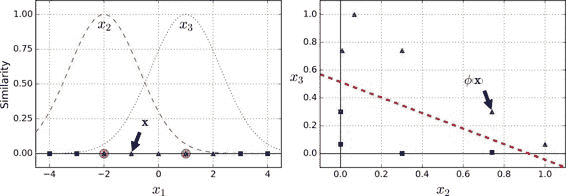### 高斯 RBF 核

rbf_kernel_svm_clf = Pipeline((
("scaler", StandardScaler()),
("svm_clf", SVC(kernel="rbf", gamma=5, C=0.001))
))
rbf_kernel_svm_clf.fit(X, y)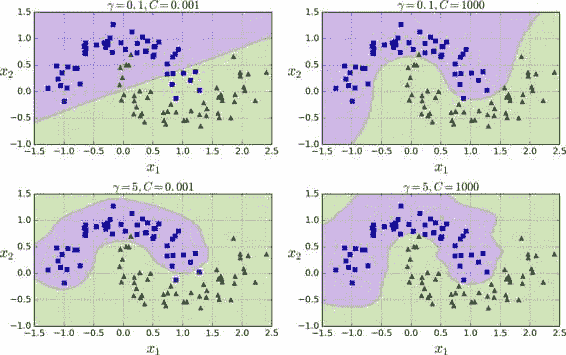### 计算复杂性

LinearSVC类基于liblinear库，它实现了线性 SVM 的优化算法。它并不支持核技巧，但是它样本和特征的数量几乎是线性的：训练时间复杂度大约为O(m × n)

SVC 类基于libsvm库，它实现了支持核技巧的算法。训练时间复杂度通常介于 O(m^2 × n)O(m^3 × n)之间。不幸的是，这意味着当训练样本变大时，它将变得极其慢（例如，成千上万个样本）。这个算法对于复杂但小型或中等数量的数据集表现是完美的。然而，它能对特征数量很好的缩放，尤其对稀疏特征来说（sparse features）（即每个样本都有一些非零特征）。在这个情况下，算法对每个样本的非零特征的平均数量进行大概的缩放。表 5-1 对 Scikit-learn 的 SVM 分类模型进行比较。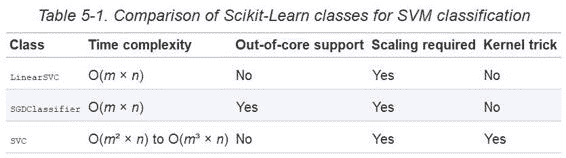## SVM 回归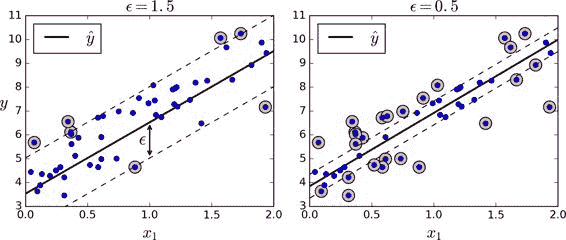from sklearn.svm import LinearSVR
svm_reg = LinearSVR(epsilon=1.5)
svm_reg.fit(X, y)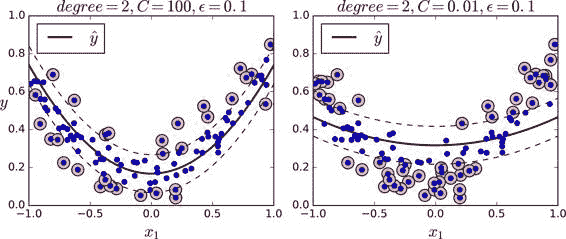from sklearn.svm import SVR

svm_poly_reg = SVR(kernel="poly", degree=2, C=100, epsilon=0.1)
svm_poly_reg.fit(X, y)


SVM 也可以用来做异常值检测，详情见 Scikit-Learn 文档

## 背后机制

### 决策函数和预测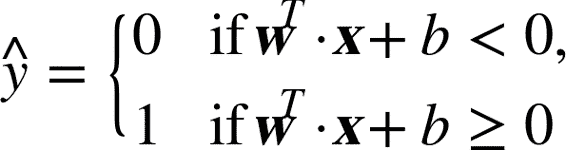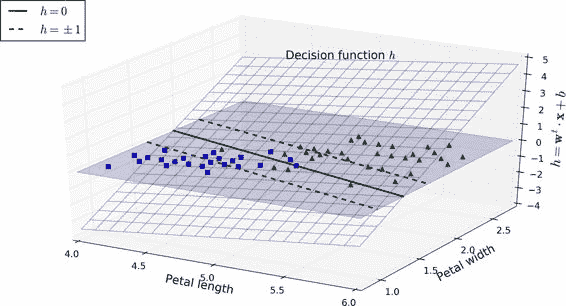### 训练目标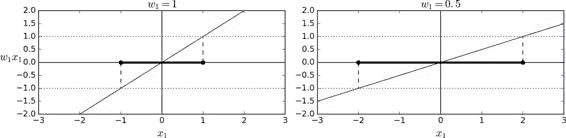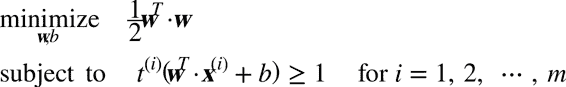1/2 w^T w等于1/2 |w|^2，我们最小化1/2 w^T w，而不是最小化|w|。这会给我们相同的结果（因为最小化w值和b值，也是最小化该值一半的平方），但是1/2 |w|^2有很好又简单的导数（只有w），|w|w=0处是不可微的。优化算法在可微函数表现得更好。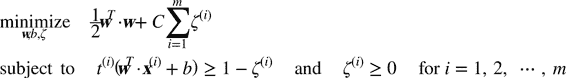### 二次规划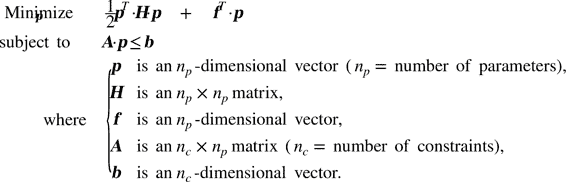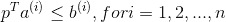a^(i)是个包含了A的第i行元素的向量，b^(i)b的第i个元素。

• n[p] = n + 1n表示特征的数量（+1 是偏置项）
• n[c] = mm表示训练样本数量
• Hn[p] * n[p]单位矩阵，除了左上角为 0（忽略偏置项）
• f = 0，一个全为 0 的n[p]维向量
• b = 1，一个全为 1 的n[c]维向量
• a^(i) = -t^(i) x_dot^(i)x_dot^(i)等于x^(i)带一个额外的偏置特征x_dot = 1

### 对偶问题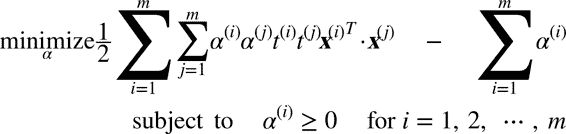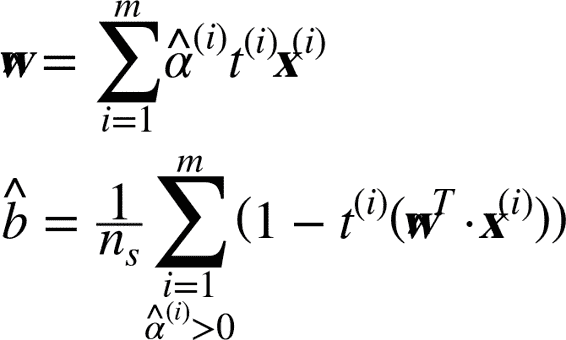### 核化支持向量机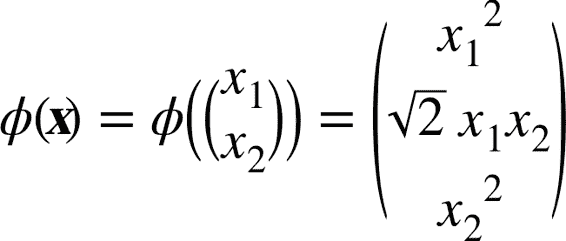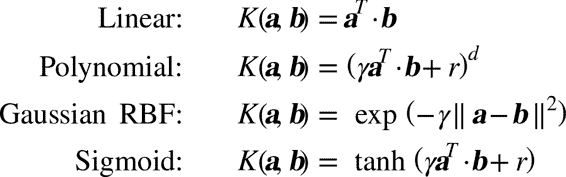Mercer 定理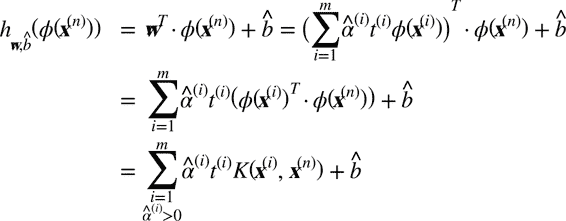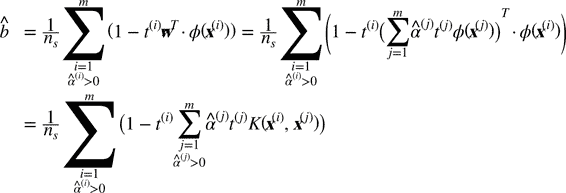### 在线支持向量机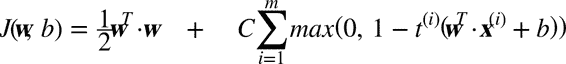Hinge 损失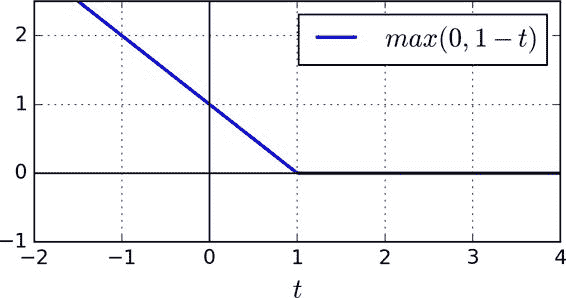## 练习

1. 支持向量机背后的基本思想是什么

2. 什么是支持向量

3. 当使用 SVM 时，为什么标准化输入很重要？

4. 分类一个样本时，SVM 分类器能够输出一个置信值吗？概率呢？

5. 在一个有数百万训练样本和数百特征的训练集上，你是否应该使用 SVM 原始形式或对偶形式来训练一个模型？

6. 假设你用 RBF 核来训练一个 SVM 分类器，如果对训练集欠拟合：你应该增大或者减小γ吗？调整参数C呢？

7. 使用现有的 QP 解决方案，你应该怎么样设置 QP 参数（HfA，和b）去解决一个软间隔线性 SVM 分类器问题？

8. 在一个线性可分的数据集训练一个LinearSVC，并在同一个数据集上训练一个SVCSGDClassifier，看它们是否产生了大致相同效果的模型。

9. 在 MNIST 数据集上训练一个 SVM 分类器。因为 SVM 分类器是二元的分类，你需要使用一对多（one-versus-all）来对 10 个数字进行分类。你可能需要使用小的验证集来调整超参数，以加快进程。最后你能达到多少准确度？

10. 在加利福尼亚住宅（California housing）数据集上训练一个 SVM 回归模型